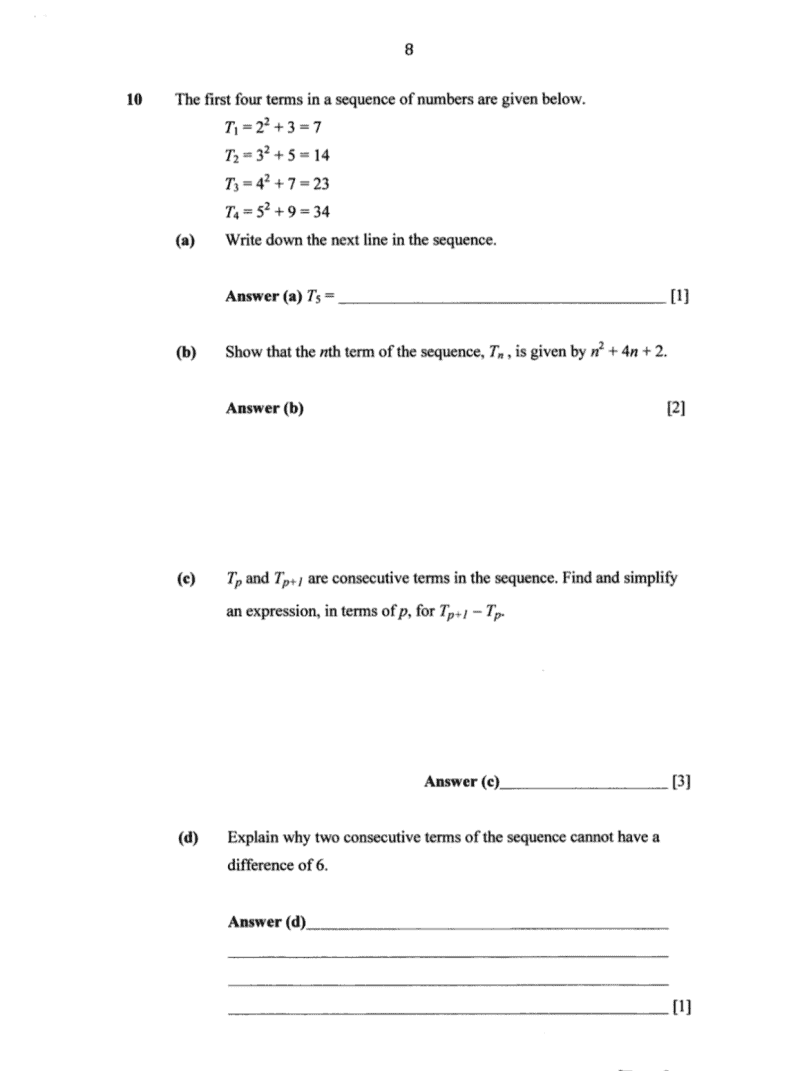# Questiona) T5 = 62 + 11

b) Taking T1 as an example, the series has 2 parts, 22 and 3.

For the 2part, since the series is increasing by 1 each time and starts at 2. It will look like this (n+1)2. We have to make sure this works for the rest as well,

For T2, for (n+1)2, (2+1)= (3)2

For T3, for (n+1)2, (3+1)= (4)2

For the 3 part, since the series is increasing by 2 each time and starts at 3. It will look like this (2n + 1). Likewise, we have to make sure this works for the rest as well,

For T2, for (2n + 1), (2(2) + 1) = 5

For T3, for (2n + 1), (2(3) + 1) = 7

Therefore the formula will look like,

Tn: (n+1)2 + (2n + 1) = (n2 +2n + 1) + (2n + 1)

= n2 +4n + 2 (hence shown)

c) Tp+1 – Tp = [(p+1)2 +4(p+1) + 2] – [p2 +4p + 2]

= [(p2+ 2p + 1) + 4p + 4 + 2] – p2 -4p – 2            {opening the brackets by multiplying the -1 inside}

= 2p-5
d) Tp+1 – Tp shows the difference between any 2 consecutive terms for all real numbers of p. The difference between any terms for all real number of p, are all odd numbers. Therefore, it is not possible for the difference between the 2 consecutive terms to be 6, as it is an even number.